Next: Bayesian methods for data Up: Practical considerations Previous: Delay coordinates in practice   Contents

## Prediction algorithms

Assume we are analysing the scalar time series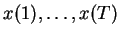. Using the time-delay embedding with delay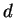transforms the series to vectors of the form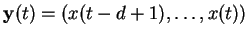. Prediction in these coordinates corresponds to predicting the next samplefrom theprevious ones, as all the other components of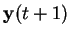are directly available in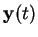. Thus the problem reduces to finding a predictor of the form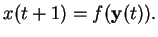(2.8)

Using a simple linear function for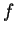leads to a linear auto-regressive (AR) model . There are also several generalisations of the same model leading to other algorithms for the same purpose.

Farmer and Sidorowich  propose a locally linear predictor in which there are several linear predictors for different areas of the embedded state-space. The achieved performance is comparable with the global linear predictor for small embedding dimensionalities but as the embedding dimension grows, the local method clearly outperforms the global one. The problem with the locally linear predictor is that it is not continuous.

Casdagli  presents a review of several different predictors including a globally linear predictor, a locally linear predictor and a global nonlinear predictor using a radial basis function (RBF) network  as the nonlinearity. According to his results, the nonlinear methods are best for small data sets whereas the locally linear method of Farmer and Sidorowich gives the best results for large data sets.

For noisy data, the choice of coordinates can make a big difference on the success of the prediction algorithm. The embedding approach is by no means the only possible alternative, and as Casdagli et al. note in , there are often clearly better alternatives. There are many approaches in the field of neural computation where the choice is left to the algorithm. This corresponds to trying to blindly invert Equation (2.7). Such models are called (nonlinear) state-space models and they are studied in detail in Section 4.2.Next: Bayesian methods for data Up: Practical considerations Previous: Delay coordinates in practice   Contents
Antti Honkela 2001-05-30